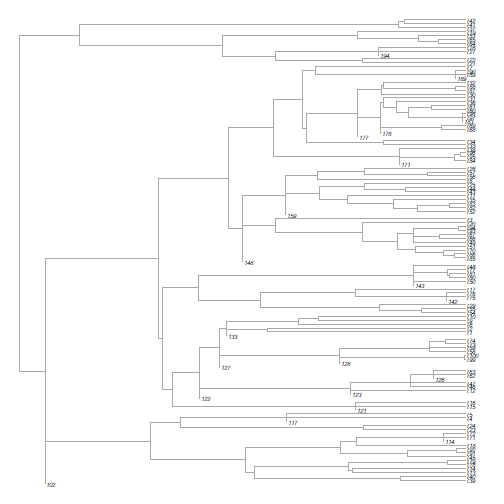## Wednesday, February 7, 2018

### Fitting continuous character models when some or all internal nodes are known

I'm working on some code to fit continuous character models in which some or all of the internal (node) states are node.

Here is the function that can (so far) fit only a simple Brownian model:

``````fitInternal<-function(tree,x,a,model="BM"){
root<-as.character(Ntip(tree)+1)
if(root%in%names(a)){
ii<-which(names(a)==root)
a0<-a[ii]
a<-a[-ii]
} else a0<-NULL
if(model=="BM"){
if(is.null(a0)){
new.root<-sample(names(a),1)
a0<-a[new.root]
a<-a[-which(names(a)==new.root)]
new.tree<-root(tree,node=as.numeric(new.root))
M<-matchNodes(tree,new.tree,"distances")
new.nodes<-sapply(as.numeric(names(a)), function(n,M)
as.character(M[which(M[,1]==n),2]),M=M)
names(a)<-new.nodes
tree<-new.tree
}
nn<-c(names(x),names(a))
V<-vcvPhylo(tree,TRUE)[nn,nn]
likBM<-function(sig2,V,y,a0)
-as.numeric(-t(y-a0)%*%solve(sig2*V)%*%(y-a0)/2-
nrow(V)*log(2*pi)/2-determinant(sig2*V)\$modulus/2)
fit<-optimize(likBM,c(0,10),V=V,y=c(x,a),a0=a0)
obj<-list(sig2=fit\$minimum,logL=-fit\$objective)
}
obj
}
``````

One of the things that I realized is that since Brownian motion is reversible, as long as I had one internal node value, I could just move the root to that node and then we'd no longer have to estimate the root node state as a second parameter in the Brownian model. Obviously, this won't work for models without this property, such as the 'early-burst' model.

Let's see how this very simple function works.

First, I'll start by simulating a tree & some data:

``````library(phytools)
tree<-pbtree(n=100)
x<-fastBM(tree,sig2=0.5,internal=TRUE)
a<-sample(x[Ntip(tree)+1:tree\$Nnode],20) ## 20 internal nodes chosen at random
x<-x[1:Ntip(tree)] ## our tip data only
``````

Now let's fit our model:

``````fit<-fitInternal(tree,x,a)
fit
``````
``````## \$sig2
##  0.4366435
##
## \$logL
##  -98.52515
``````

As I've documented in the past, another way to fit this model is by adding terminal edges of zero length to each node for which we have data. Let's do this to cross-check our result:

``````nodes<-as.numeric(names(a))
tt<-tree
for(i in 1:length(nodes)){
M<-matchNodes(tree,tt,"distances")
node<-M[which(M[,1]==nodes[i]),2]
tt<-bind.tip(tt,nodes[i],edge.length=0,where=node)
}
plotTree(tt,fsize=0.5,lwd=1,color="darkgrey",ftype='i')
````````````fitContinuous(multi2di(tt),c(x,a))
``````
``````## GEIGER-fitted comparative model of continuous data
##  fitted 'BM' model parameters:
##  sigsq = 0.433003
##  z0 = 0.180955
##
##  model summary:
##  log-likelihood = -98.214025
##  AIC = 200.428050
##  AICc = 200.530614
##  free parameters = 2
##
## Convergence diagnostics:
##  optimization iterations = 100
##  failed iterations = 0
##  frequency of best fit = 1.00
##
##  object summary:
##  'lik' -- likelihood function
##  'bnd' -- bounds for likelihood search
##  'res' -- optimization iteration summary
##  'opt' -- maximum likelihood parameter estimates
``````

These are almost the same - but not quite. It turns out that our first estimate, though estimed using ML, is identical (to a high degree of numerical precision) to the REML estimation of σ2. We can show that using the phytools function `brownieREML` as follows:

``````tt<-paintSubTree(multi2di(tt),Ntip(tt)+1,"1")
fitREML<-brownieREML(tt,c(x,a))
fitREML
``````
``````## REML single-rate model:
##  s^2 se  k   logL
## value    0.4366  0.0566  1   -98.5251
##
## REML multi-rate model:
##  s^2(1)  se(1)   k   logL
## value    0.4366  0.0566  1   -98.5251
##
## R thinks it has found the REML solution.
``````
``````fitREML\$sig2.single
``````
``````##  0.4366413
``````
``````fit\$sig2
``````
``````##  0.4366435
``````

Nonetheless, the purpose of doing this is to fit more complicated models such as OU or EB, so I will plan to add this later.